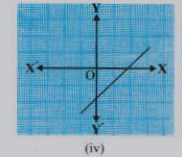# In general, given a polynomial p(x) of degree n, the graph of y = p(x) intersects the x-axis atmost n points. Therefore, a polynomial p(x) of degree n has atmost n zeroes:Solution in Punjabi

Updated on:21/07/2023